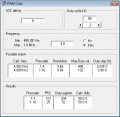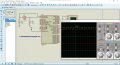# Wanted to generate PWM pulses using PIC16F87XA

Thread Starter

#### khatus

Joined Jul 2, 2018
89
Hello guys I wanted to generate 4.9kHZ Pwm using Pic micro controller with out using in build functions.I am using PIC16F87XA mcu this time .
Here is my code
Code:
void main()
{
PR2=0x65; // Frequency: 4.90 kHz
CCP1CON=0b00001100;   //Configure the CCP1 module
T2CON.T2CKPS0 = 1;
T2CON.T2CKPS1 = 0;
TRISC.B2 = 0; // make port pin on C as output
while(T2CON.TMR2ON = 1) //Infinite Loop
{
}
}
Here is the steps that i have followedBUT while simulating it in proteus i did not get any result from RC2 PIN(CCP1 pin of PC microcontroller) i did not get any pulse from the pin.
What is the problem??

#### hexreader

Joined Apr 16, 2011
532

#### sagor

Joined Mar 10, 2019
439
You are not setting a value in CCPR1L nor CCPCON1 <5:4> for duty cycle. So, with a 0% duty cycle (no bits set), your PWM cannot output anything.
PWM Duty Cycle =(CCPR1L:CCP1CON<5:4>) * TOSC * (TMR2 Prescale Value)

What speed is the PIC running at? That determines the PR2 values and others... The 16F87xA datasheet I have shows example for 20Mhz, with totally different values than yours. A simple PWM calculator shows: (PR2 = 101 decimal which is 0x65)#### chayanforyou

Joined Nov 12, 2015
1
I have already answered
https://www.eevblog.com/forum/beginners/wanted-to-generate-pwm-pulses-using-ic16f87xa/
https://forum.mikroe.com/viewtopic.php?f=88&t=76413

C-like:
/*

PWM Generation 4.88KHz

********************************

Compiler: mikroC Pro for PIC

For
PIC 16F877A @ 20MHz

CONFIG:
\$2007 : 0x2F42

Copyright (c) 2020
Chayan Mistry

*/

// --- Function for Setting PWM Duty Cycle ---
void SetPWMDutyCycle(unsigned int DutyCycle) // Give a value in between 0 and 1024 for DutyCycle
{
CCPR1L   = DutyCycle>>2;                // Put MSB 8 bits in CCPR1L
CCP1CON &= 0xCF;                        // Make bit4 and 5 zero
CCP1CON |= (0x30&(DutyCycle<<4));       // Assign Last 2 LSBs to CCP1CON
}

// --- Main Function ---
void main()
{
TRISC.B2  = 0;          // Make CCP1 pin as output
CCP1CON = 0x0C;         // Configure CCP1 module in PWM mode

PR2   = 0xFF;           // Configure the Timer2 period
T2CON = 0x01;           // Set Prescaler to be 4, hence PWM frequency is set to 4.88KHz.

SetPWMDutyCycle(512);   // Intialize the PWM to 50% duty cycle

T2CON |= 0x04;          // Enable the Timer2, hence enable the PWM.

while (1)               //Infinite loop
{

} //end while

} //end mainLast edited:
Thread Starter

#### khatus

Joined Jul 2, 2018
89
Here is my code This time i wanted to vary pwm signal according to a potentiometer connected in pin A1.Here is my code for mikroc.But THe pwm signal did not vary according to the adc value.
Code:
void PWM_Init(unsigned char period)
{
TRISC &=0xFD;  //  Set RC2 as output

/* CCP PWM mode */
CCP1CON &= 0xCF;  //  5,4 bits zeroed (DC1B1:DC1B0 = 00)
CCP1CON |= 0x0C;  //  PWM mode ( CCP1M3:CCP1M0 = 1100)

/* Timer2 configuration */
PR2 = period;  //  configure timer2 period
T2CON = 0x02;  //  Set prescalar 16
TMR2ON_bit = 1;  //  timer2 on

}
void PWM_setDC(unsigned int dutycycle)
{

CCPR1L = dutycycle>>2;  //  PWM duty cycle - first 8-bits (MSb)
CCP1CON &= 0xCF;  //  5,4 bits zeroed (DC1B1:DC1B0 = 00)
CCP1CON |= ((dutycycle<<4)&0x30);  //  PWM duty cycle - last 2-bits (LSb) in CCP1CON 5,4 bits
}
void main()
{
int adc_value;
TRISC = 0x00; //PORTC as output
TRISA = 0xFF; //PORTA as input
PWM_Init(0xFF);
//This sets the PWM frequency of PWM1

while(1); //Infinite Loop
{

adc_value = ADC_Read(1); //Reading Analog Channel 0
PWM_setDC(adc_value);
delay_ms(50);
}
}https://openlabpro.com/guide/pulse-...nlGO5xAImaFrZUSOzNqZa5DS4t4Hts6kWZaBuF_nyQBIg
I used the following link code for my code.

#### MaxHeadRoom

Joined Jul 18, 2013
23,104
Similar threads